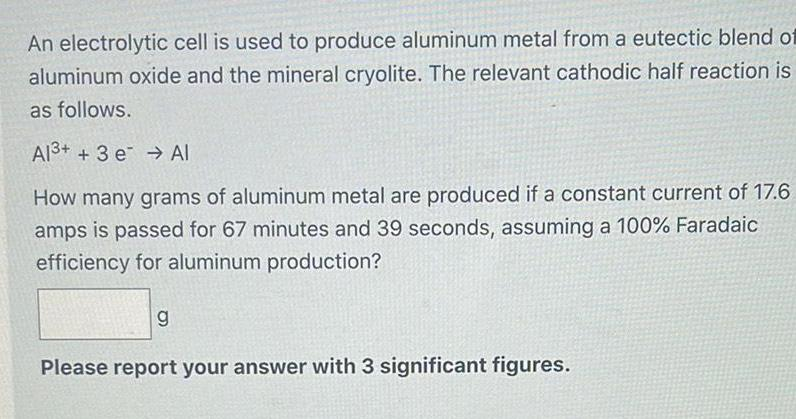Physical Chemistry
Electrochemistry
An electrolytic cell is used to produce aluminum metal from a eutectic blend of aluminum oxide and the mineral cryolite. The relevant cathodic half reaction is as follows. Al³+ + 3 e Al How many grams of aluminum metal are produced if a constant current of 17.6 amps is passed for 67 minutes and 39 seconds, assuming a 100% Faradaic efficiency for aluminum production? Please report your answer with 3 significant figures.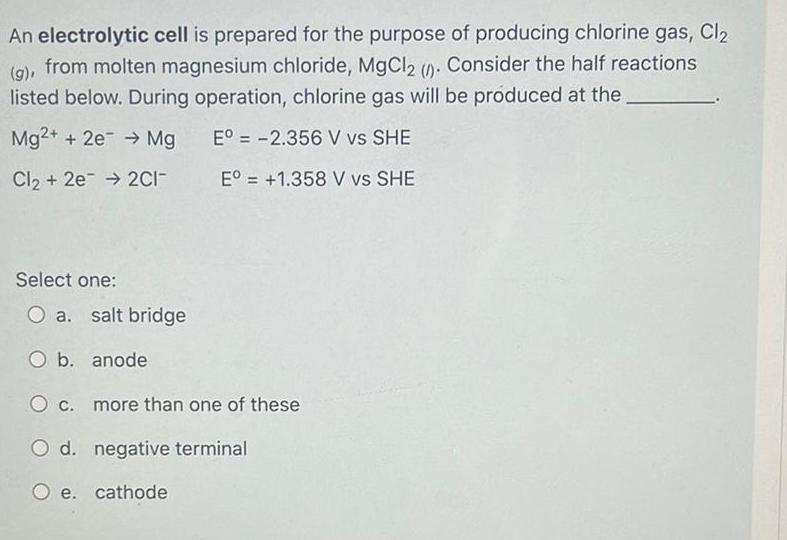Physical Chemistry
Electrochemistry
An electrolytic cell is prepared for the purpose of producing chlorine gas, Cl₂ (g), from molten magnesium chloride, MgCl2 (1). Consider the half reactions listed below. During operation, chlorine gas will be produced at the. Mg2+ + 2e → Mg Eº = -2.356 V vs SHE Cl₂ + 2e → 2C1- Eº = +1.358 V vs SHE Select one: O a. salt bridge O b. anode O c. more than one of these O d. negative terminal O e. cathode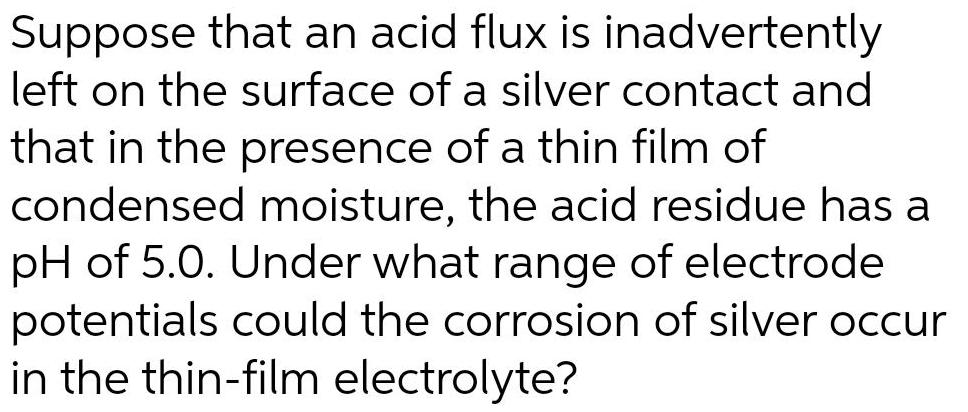Physical Chemistry
Electrochemistry
Suppose that an acid flux is inadvertently left on the surface of a silver contact and that in the presence of a thin film of condensed moisture, the acid residue has a pH of 5.0. Under what range of electrode potentials could the corrosion of silver occur in the thin-film electrolyte?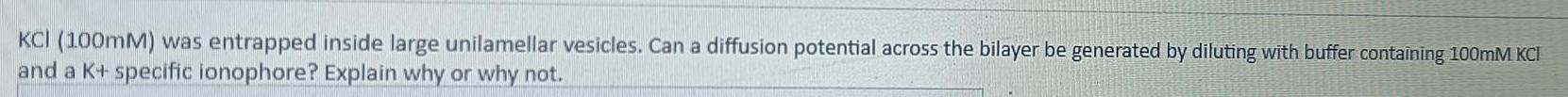Physical Chemistry
Electrochemistry
KCI (100mM) was entrapped inside large unilamellar vesicles. Can a diffusion potential across the bilayer be generated by diluting with buffer containing 100mM KCI and a K+ specific ionophore? Explain why or why not.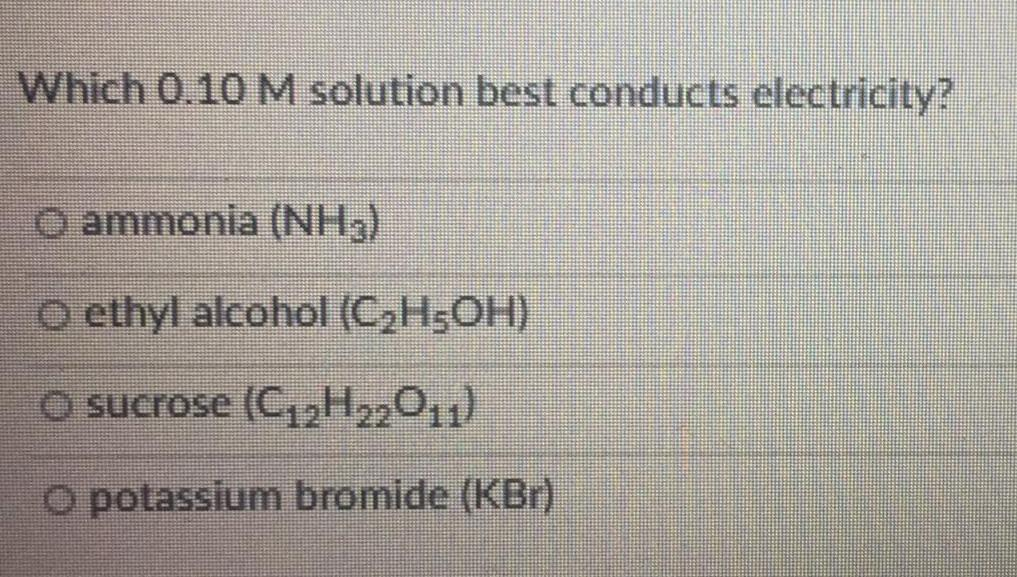Physical Chemistry
Electrochemistry
Which 0.10 M solution best conducts electricity? ammonia (NH3) ethyl alcohol (C₂H5OH) sucrose (C₁₂H22011) potassium bromide (KBr)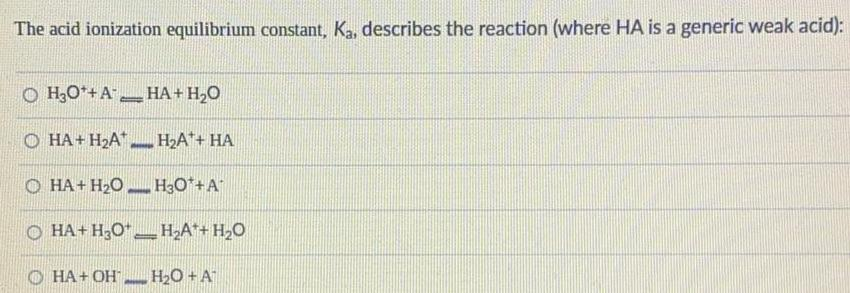Physical Chemistry
Electrochemistry
The acid ionization equilibrium constant, Ka, describes the reaction (where HA is a generic weak acid): H3O+A- <--> HA+H₂O HA+H₂A+ <--> H₂A++ HA HA+ H₂O <--> H3O++A- HA+H3O+ <--> H₂A++ H₂O HA+OH- <--> H₂O + A-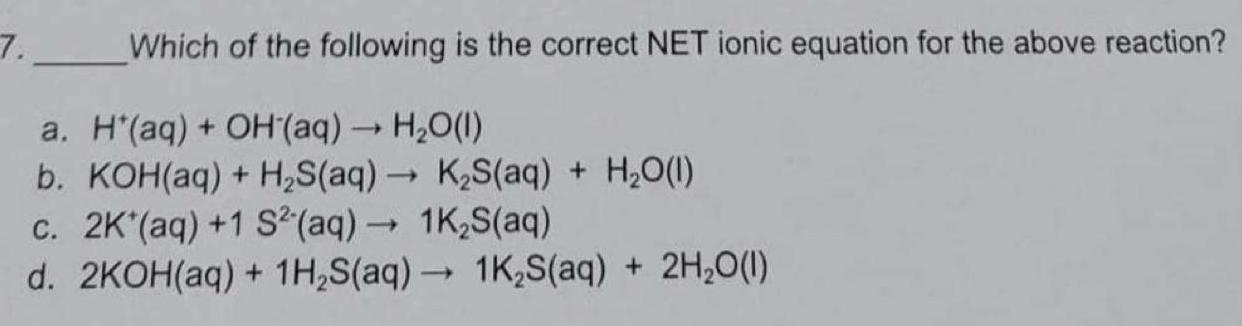Physical Chemistry
Electrochemistry
Which of the following is the correct NET ionic equation for the above reaction? a. H+(aq) + OH(aq) → H₂O(l) b. KOH(aq) + H₂S(aq) → K₂S(aq) + H₂O(l) c. 2K+(aq) +1 S²-(aq) → 1K₂S(aq) d. 2KOH(aq) + 1H₂S(aq) → 1K₂S(aq) + 2H₂O(l)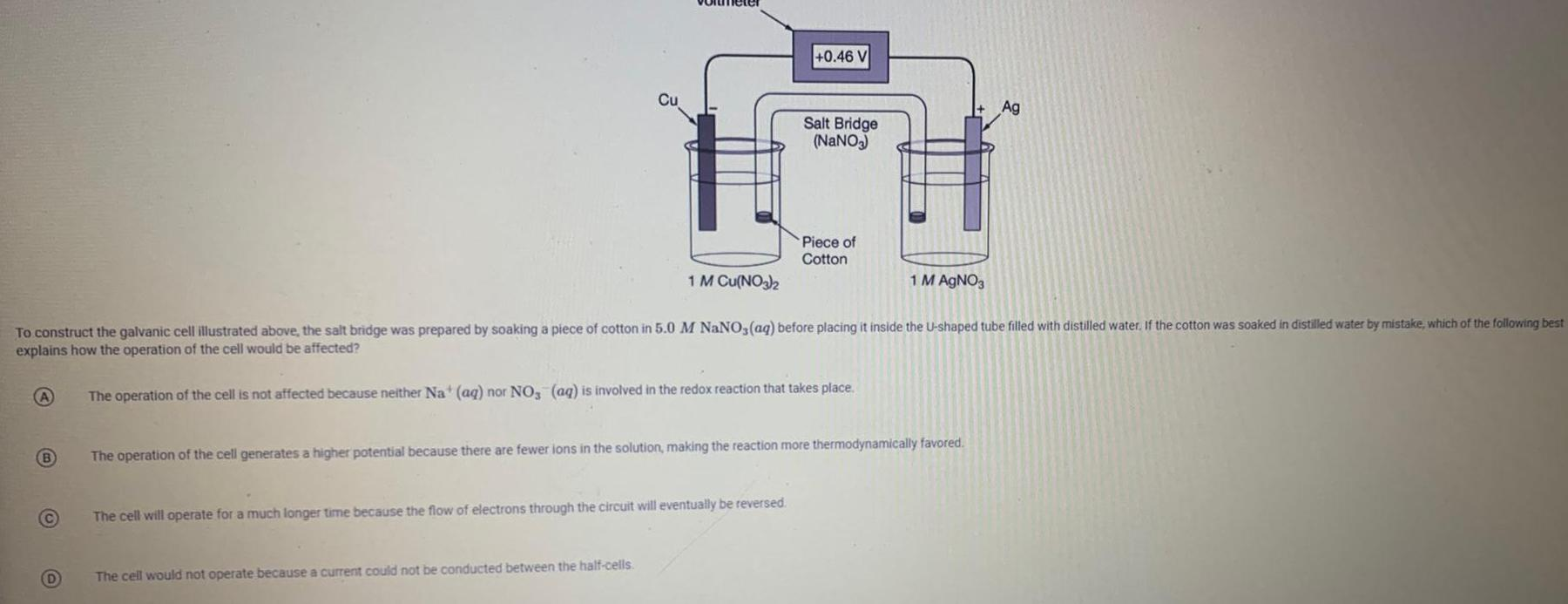Physical Chemistry
Electrochemistry
B C D Cu 1 M Cu(NO3)2 The cell would not operate because a current could not be conducted between the half-cells. +0.46 V To construct the galvanic cell illustrated above, the salt bridge was prepared by soaking a piece of cotton in 5.0 M NaNO3(aq) before placing it inside the U-shaped tube filled with distilled water. If the cotton was soaked in distilled water by mistake, which of the following best explains how the operation of the cell would be affected? The operation of the cell is not affected because neither Na+ (aq) nor NO3(aq) is involved in the redox reaction that takes place. The cell will operate for a much longer time because the flow of electrons through the circuit will eventually be reversed. Salt Bridge (NaNO3) Piece of Cotton 1 M AgNO3 The operation of the cell generates a higher potential because there are fewer ions in the solution, making the reaction more thermodynamically favored. Ag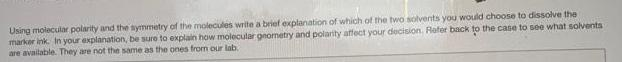Physical Chemistry
Electrochemistry
Using molecular polarity and the symmetry of the molecules write a brief explanation of which of the two solvents you would choose to dissolve the marker ink. In your explanation, be sure to explain how molecular geometry and polarity affect your decision. Refer back to the case to see what solvents are available. They are not the same as the ones from our lab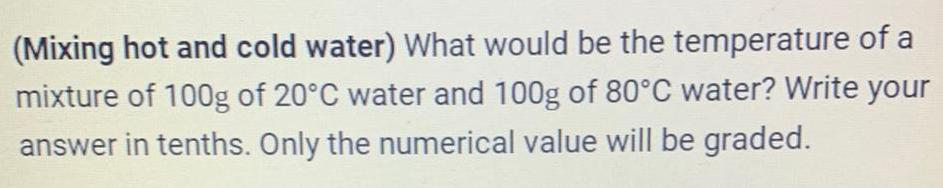Physical Chemistry
Electrochemistry
(Mixing hot and cold water) What would be the temperature of a mixture of 100g of 20°C water and 100g of 80°C water? Write your answer in tenths. Only the numerical value will be graded.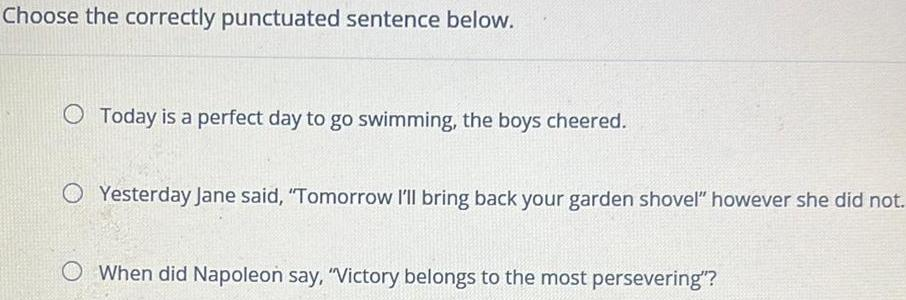Physical Chemistry
Electrochemistry
Choose the correctly punctuated sentence below. Today is a perfect day to go swimming, the boys cheered. Yesterday Jane said, "Tomorrow I'll bring back your garden shovel" however she did not. When did Napoleon say, "Victory belongs to the most persevering"?Physical Chemistry
Electrochemistry
Choose the correct answer for the following question from the choices below. Which option contains a sentence that is punctuated correctly? O "Heather called out, Are you finished? We really should get going." Heather called out "Are you finished?" We really should get going. Heather called out, "Are you finished? We really should get going."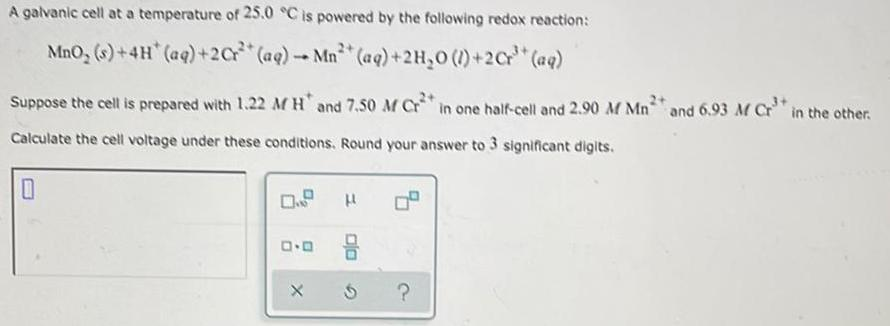Physical Chemistry
Electrochemistry
A galvanic cell at a temperature of 25.0 °C is powered by the following redox reaction: MnO₂ (s)+4H* (aq) +2Cr²+ (aq) - Mn³ (aq) + 2H₂O (1)+2Cr³+ (aq) 1 Suppose the cell is prepared with 1.22 M H and 7.50 M Cr2+ in one half-cell and 2.90 M Mn2 and 6.93 M Cr³+ in the other. Calculate the cell voltage under these conditions. Round your answer to 3 significant digits. 0 D.D H 010 ?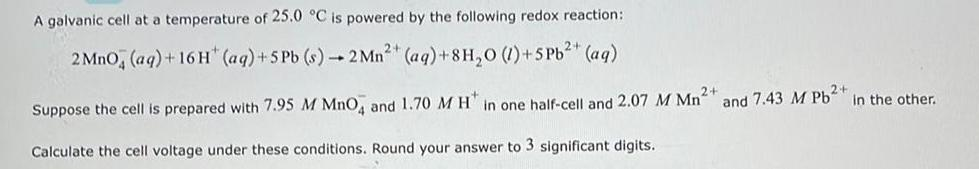Physical Chemistry
Electrochemistry
A galvanic cell at a temperature of 25.0 °C is powered by the following redox reaction: 2 MnO, (aq) + 16H* (aq) +5 Pb (s)-2 Mn²+ (aq) +8H₂0 (1) +5 Pb²+ (aq) Suppose the cell is prepared with 7.95 M MnO4 and 1.70 M H in one half-cell and 2.07 M Mn²+ Calculate the cell voltage under these conditions. Round your answer to 3 significant digits. and 7.43 M Pb2+ in the other.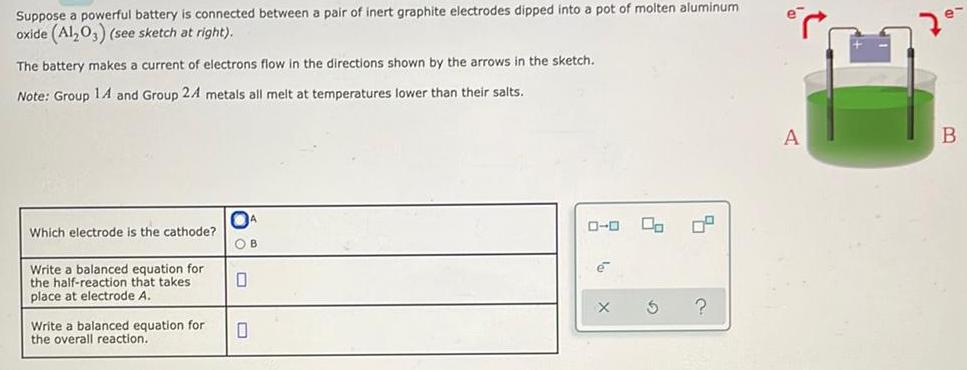Physical Chemistry
Electrochemistry
Suppose a powerful battery is connected between a pair of inert graphite electrodes dipped into a pot of molten aluminum oxide (Al2O3) (see sketch at right). The battery makes a current of electrons flow in the directions shown by the arrows in the sketch. Note: Group 14 and Group 24 metals all melt at temperatures lower than their salts. Which electrode is the cathode? Write a balanced equation for the half-reaction that takes place at electrode A. Write a balanced equation for the overall reaction. A OB 0 0 0-0 la X G ? A H 7e- B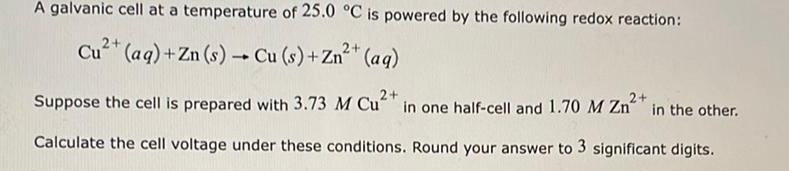Physical Chemistry
Electrochemistry
A galvanic cell at a temperature of 25.0 °C is powered by the following redox reaction: 2+ Cu²+ (aq) + Zn (s) → Cu (s) + Zn²+ (aq) - 2+ Suppose the cell is prepared with 3.73 M Cu2+ in one half-cell and 1.70 M Zn²+ in the other. Calculate the cell voltage under these conditions. Round your answer to 3 significant digits.